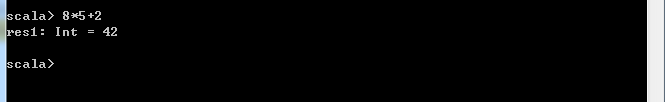# Scala入门

2021-05-15 63点热度 0人点赞 0条评论## 1. scala的由来

scala是一门多范式的编程语言，一种类似java的编程语言 ，设计初衷是要集成面向对象编程和函数式编程的各种特性。

java和c++的进化速度已经大不如从前，那么乐于使用更现代的语言特性的程序员们正在将眼光移向他处。scala是一个很有吸引力的选择；事实上，在我看来，对于想要突破和超越java或者c++的程序员而言，scala是最具吸引力的一个。scala的语法十分简洁，相比java的样板代码，scala让人耳目一新。scala运行于java虚拟机之上，让我们可以使用现成的海量类库和工具。他在拥抱函数式编程的同时，并没有非其面向对象，使你得以逐步了解和学习一种全新的编程范式。scala解释器可以让你快速运行试验代码，这使得学习scala的过程颇为轻松惬意。最后，同时也是很重要的一点，scala是静态类型的，编译器能够帮助我们找到大部分错误，避免时间的浪费。

## 2.Scala基础

2.1. scala****环境变量安装

2.2. 认识****scala2.3. 安装****scala-eclipse

2.3.1. 安装

2.3.2. 打开2.3.3. 创建****scala****工程2.3.4. 创建****scala****包2.3.5. 创建****scala****类2.4. 创建变量

Scala 定义了两种类型的变量 val 和 var ，val 类似于Java中的final 变量，一旦初始化之后，不可以重新赋值（我们可以称它为常变量）。而var 类似于一般的非final变量。可以任意重新赋值。

2.4.1. 不指定类型

package org.apache.first

object test {

def main(args: Array[String]): Unit = {
val myint = 1
val mystring ="string"
var myint2 = 1
var mystring2 ="string"
println(myint)
println(mystring)
println(myint2)
println(mystring2)
}

}


2.4.2. 指定类型

val myint:Int = 1;
val mystring:String =null;


2.4.3. 常用变量

1.toString(); //产生字符串“1”

​ 1.to(10) //产生Range(1, 2, 3, 4, 5, 6, 7, 8, 9, 10)，类似数组

2.5. 算数和操作符重载

a+b

a.+(b)

2.6. 定义函数

Scala既是面向对象的编程语言，也是面向函数的编程语言，因此函数在Scala语言中的地位和类是同等第一位的。下面的代码定义了一个简单的无返回值和有返回值的代码：

2.6.1. 空参无返回值

def main(args: Array[String]): Unit = {

option()

}

def option(): Unit = {

println("ok")

}


option函数的返回值类型为Unit 表示该函数不返回任何有意义的值，Unit类似于Java中的void类型

2.6.2. 空参有返回值

def main(args: Array[String]): Unit = {
val returnval = option()
println(returnval);
}
def option(): Int = {
1
}


2.6.3. 有参数有返回值

def main(args: Array[String]): Unit = {
val returnval = option(3,5)
println(returnval);
}
def option(x:Int,y:Int): Int = {
if (x >y) x
else
y
}


Scala函数以def定义，然后是函数的名称（如option)，然后是以逗号分隔的参数。Scala中变量类型是放在参数和变量的后面，以“：”隔开。同样如果函数需要返回值，它的类型也是定义在参数的后面（实际上每个Scala函数都有返回值，只是有些返回值类型为Unit，类似为void类型）。

3. 控制结构和函数

3.1. 条件表达式****if else

scala的if/else语法结构和java一样。不过，在scala中if/else表达式有值，这个值就跟在if或者else之后的表达式值。例如：

if(x>0) 1 else -1


val s = if (x>0) 1 else -1


val x = 0

var s =0

if (x>0) {

s=1

}else{

s=2

}


3.2. 语句终止

3.3. 块表达式和赋值

package org.apache.first

import scala.math._

object test {

def main(args: Array[String]): Unit = {

val test = {
val dx = 0;
val dy = 10;
min(dx,dy);
}
println(test)
}


3.4. 循环
3.4.1. while循环

object test {

def main(args: Array[String]): Unit = {

var n = 10;

while(n>0){

println(n)

n-=1;

}

}


3.4.2. for

def main(args: Array[String]): Unit = {
for(i <- 1 to 10){
println(i)
}
}


import scala.util.control.Breaks._
object test {
def main(args: Array[String]): Unit = {
for(i <- 1 to 10){
if(i==3) return;
println(i)
}
}


3.4.3. 高级for循环

object test {
def main(args: Array[String]): Unit = {
for(i <- 1 to 10;j <- 1 to 3){

println(i+j)

}

}

object test {

def main(args: Array[String]): Unit = {

for(i <- 1 to 10;j <- 1 to 3 **if** i == j){

println(i+j)

}
}


3.5. 函数

3.5.1. 默认参数

object test {

def main(args: Array[String]): Unit = {

val aa = option(2);

println(aa);

}

def option(x:Int,y:Int=1): Int = {

if (x >y) x

else
y
}

}


option函数有两个参数，但是y这个参数是带默认值的，所以，如果你只传一个参数x，那么y就会走默认值。

3.5.2. 变长参数

object test {

def main(args: Array[String]): Unit = {
option(2,3,4,5,6);
}

def option(args:Int*): Unit = {
println(args(3))
for(arg <- args){
println(arg);
}
}
}


3.5.3. 递归

object test {
def main(args: Array[String]): Unit = {
var aa = option(2,3,4,5,6);
println(aa)
}

def option(args:Int*): Int = {

if(args.length==0){
0
}else{

args.head + option(args.tail: _*)
}
}

}


3.6. 过程

scala对于不返回值的函数有特殊的表示法。如果函数体包含在花括号当中，但是没有等号“=”，那么返回值类型就是Uint。这样的函数被称为过程（procedure）。过程不返回任何值。

object test {

def main(args: Array[String]): Unit = {
option(2);
}
def option(x:Int) = {

println(x+5)
}
}


3.7. 懒值

def main(args: Array[String]): Unit = {

lazy val words = scala.io.Source.fromFile("/usr/local/a.txt").mkString;
//println(words);
}


3.8. 异常

scala的异常工作机制和java的一样。当你抛出异常时，比如：

throw new IllegalArgumentException("aaa");


try{

}catch{

}finally{

}


4. 数组及操作

4.1. 定长数组

//3个整数的数据，所有元素为0

val arr1 = new Array[Int](3)

val arr2 = Array(0, 0, 0)

val arr3 = Array("hadoop", "spark")

//取值、赋值

val str = arr3(1)

arr3(1) = "storm"


4.2. 变长数组
ArrayBuffer类似Java中的ArrayList

val a = ArrayBuffer[Int]()

a +=1 //在尾端添加元素

a +=(1,2,3) //追加多个元素

a ++= ArrayBuffer(7,8,9) //追加集合

a.trimEnd(2) //移除最好两个元素

a.insert(2, 6, 7) //在下标2之前插入6，7

a.remove(2, 2) //从下标2开始移除2个元素

val b = a.toArray //转变成定长数据组

b.toBuffer //转变成变长数据组


4.3. 遍历数组

val arr = Array(1,2,3,4,5)

//带下标的for循环

for (i <- (0 until (arr.length, 2)).reverse) {     println(arr(i))

}

//增强for循环

for (i <- arr) println(i)


4.4. 数组转换

val arr = Array(1,2,3,4,5,6,7,8,9,10)

for(e <- arr) yield e //生成一个与arr一样的

for(e <- arr if e % 2 == 0) yield e * 2

//思考：如何变得跟简单


4.5. 数组的常用算法

val arr = Array(4,3,5,1,2)

val res = arr.sum

Array("spark","hadoop", "storm").min

val b = arr.sorted

val c = arr.sortWith(_>_)

val d = arr.count(_>2)


5. 映射

val map = Map("a"->1, "b"->2, "c"->3)

//取值、赋值

map("a")

map.getOrElse("d", 0)

map("b") = 22

map += ("e"->8, "f"->9)

map -= ("f")

//迭代

for((k,v) <- map)

//交换k,v

for((k,v) <- map) yield (v,k)

//keySet和values


6. 元祖

val t = ("hadoop", 1000, "spark")

val a = t._3 //元组中的下标是从1开始
"NewYork".partition(_.isUpper)


7. 复杂的集合操作方法

7.1.1. map

val a = Array(6,7,8,9)


a.map(_*2)// Array[Int] = Array(12, 14, 16, 18)


map((_,1))// Array((6,1), (7,1), (8,1), (9,1))

val map = Map("a"->1, "b"->2, "c"->3)

map.map(x=>x._1)//取出map的第一个值

map.map(x=>(x._1,x._2))//取出map的所有值


7.1.2. flatten

val a = Array(Array(6,7,8,9), Array(10,11,12,13,14))

val res = a.faltten// val a = Array(Array(6,7,8,9), Array(10,11,12,13,14))


7.1.3. groupby

val map = Map("a"->1, "b"->2, "c"->3)


7.1.4. foldLeft

val a = Array(1,2,3,4,5)
a.foldLeft(0)(_+_) //15


7.1.5. reduce

val a = Array(1,2,3,4,5)
a.reduce(_+_) //15


7.1.6. aggregate

aggregate函数将每个分区里面的元素进行聚合，然后用combine函数将每个分区的结果和初始值(zeroValue)进行combine操作。这个函数最终返回的类型不需要和RDD中元素类型一致。

val a = Array(Array(1,2,3),Array(4,2,3))

1. aggregate(0)(_+_.sum,_+_)


7.1.7. flatMap

7.1.8. reduceByKey

7.1.9. sortByKey

7.1.10. sortBy

8.

8.1. 类的定义和****getset****方法

var

val

private和private[this]

8.2. 主构造器和辅助构造器

1、跟在类名后面的是主构造器

2、辅助构造器的名称为this定义，必须执行def

3、辅助构造函数相当于java中的其他的累的构造器，所接受的参数不能多于主类的

class TestScala1 (val name: String,var password:String ) {

​     def this(name: String) = this(name,null)

}


8.3. object****半生对象

8.4.

8.5. 模式匹配

Scala有一个非常强大的模式匹配机制，可以应用到很多场合：如switch、类型查询，并可以结合样例类完成高级功能

object TestScala1 { def main(args: Array[String]): Unit = {  val aa = new TestScala2  println(aa.test("zhangsan")) } }class TestScala2 { def test(name: String): String = {  //和java的switch一样，只是关键字变成了match,分在=》后面的是一个方法体，而=》符号表示匿名函数  name match {   case "zhangsan" => TestScala2.test(name)   case "lisi" => name+"fdsfas"   case _ => name+"fdsfas"   } }}object TestScala2 {     def test(name: String): String = {              "wangwu"     }}


8.6. 样本类

**case** **class** TestScala1(**val** name: String, **var** password: String) { **def** **this**(name: String) = **this**(name, **null**) **def** **this**() = **this**(**null**, **null**)}


样本类：添加了case的类便是样本类。这种修饰符可以让Scala编译器自动为这个类添加一些语法上的便捷设定。如下：

9. 继承

o.isInstanceOf[Cl] //java中的instanceof

o.asInstacneOf[Cl] //java中的 (Cl) o

classOf[Cl] //java中的Cl.class


10. 高阶函数

def fun(f: Double => Double) = {
f(100)
}

fun((x : Double) => 2 * x)

fun(sqrt _)


11. 柯里化

def first(x:Int) = (y:Int) => x + y


val second=first(1)
val result=second(2)


def fun(x: Int)(y: Int) = x * y


fun(6)(7)


val fun2 = fun(6)_  // 这次调用返回的是一个方法，传入一个值，但是本身这个函数需要两个值，所以我们用”_”来站位我们不知道的另一个参数

val result = fun2(7)  // 42


12. 隐式转换

1. 例如：java的file类中并没有read方法，那么我们想读文件，需要利用scala的source这个类帮我们读文件
2. 那么，我们重新定义一个类RichFile，接受的参数是File类型，而利用Source.fromFile(file.getPath()).mkString帮助我们读取文件，但是这个方法位于RichFile中，无法让File类直接使用，所以我们需要将File转换成RichFile
3. 那么，我们写一个object伴生对象，将我们的File转换成RichFile，书写如下，注意必须有impilicit关键字声明方法：implicit def file2RichFile(f:File)= new RichFile(f)
4. 在我们需要使用File调用read方法时，import之前的半生对象即可import Context._
package org.apache.scala.one
import scala.collection.mutable.Mapimport scala.math._
import scala.io.Source
import java.io.File
class RichFile(val file:File){
def read = Source.fromFile(file.getPath()).mkString}
object Context{  implicit def file2RichFile(f:File)= new RichFile(f)}

object ImplicitDemo extends App{
import Context._
println(new File("c:/sss.txt").read)
1.to(10)
}